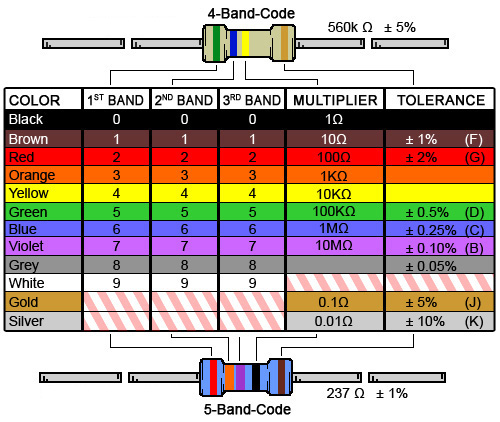Welcome to Project Lovelace! We're still in early development so there are still tons of bugs to find and improvements to make. If you have any suggestions, complaints, or comments please let us know on Discourse, Discord, or GitHub!

# Colorful resistors

## You will learn about: electrical components and dictionaries.

Let's use dictionaries (you don't have to of course) to look at resistors, which are common electric components found in virtually every electronic circuit. Resistors come with a resistance rating $\;\Omega$ (measured in Ohms) that describes how difficult it is to pass an electric current through the resistor. This resistance rating is indicated by four or five colored bands on the resistor itself (see table). A resistor may have only one band, in which case it is a single blank band and the resistor has zero resistance.Resistor color table with example calculations for a four-band and a five-band resistor. (Image credit: Resistors and Color Codes, Digi-Key)

For a four band resistor, the first two color bands indicate the first two digits of the resistance value with the third band indicating a multiplier, and the last band indicates a tolerance. For a five band resistor, the first three color bands indicate the first three digits of the resistance value followed by a fourth band (multiplier) and a fifth band (tolerance). Keep in mind that the tolerance is a relative tolerance, like ±5%.

Using a list of colors as input compute the nominal resistance (i.e. as told by the colored bands, whithout any tolerance), as well as the minimum and maximum resistance value of the resistor, and return them in that order.

Input: A list of colors (could be of length 1, 4, or 5).

Output: The nominal, minimum, and maximum resistance of the resistor (in that order).

Example 1 (four-band resistor)

Input resistor band colors: ["green", "blue", "yellow", "gold"] Output nominal resistance: 560000 Output minimum resistance: 532000 Output maximum resistance: 588000

Example 2 (five-band resistor)

Input resistor band colors: ["red", "orange", "violet", "black", "brown"] Output nominal resistance: 237.00 Output minimum resistance: 234.63 Output maximum resistance: 239.37
 Difficulty resistance(band_colors)

You must be logged in to view your submissions.

### Notes

• Since $1\;\Omega$ is a very small resistance, it's more common to express resistance in kilohms ($1\;\text{k}\Omega = 1,000\;\Omega$), megaohms ($1\;\text{M}\Omega = 1,000,000\;\Omega$), or even gigaohms ($1\;\text{G}\Omega = 1,000,000,000\;\Omega$).
• Nowadays it's easier to use a multimeter to measure it's actual resistance value. Calculating a resistor's value and tolerance from the color bands used to be more commonplace in the past when multimeters weren't as widespread or cheap.
• If you need a very specific resistance you can chain resistors in series or parallel. Variable resistors also exist in the form of potentiometers and digital resistors.

Let us know what you think about this problem! Was it too hard? Difficult to understand? Also feel free to discuss the problem, ask questions, and post cool stuff on Discourse. You should be able see a discussion thread below. Would be nice if you don't post solutions in there but if you do then please organize and document your code well so others can learn from it.